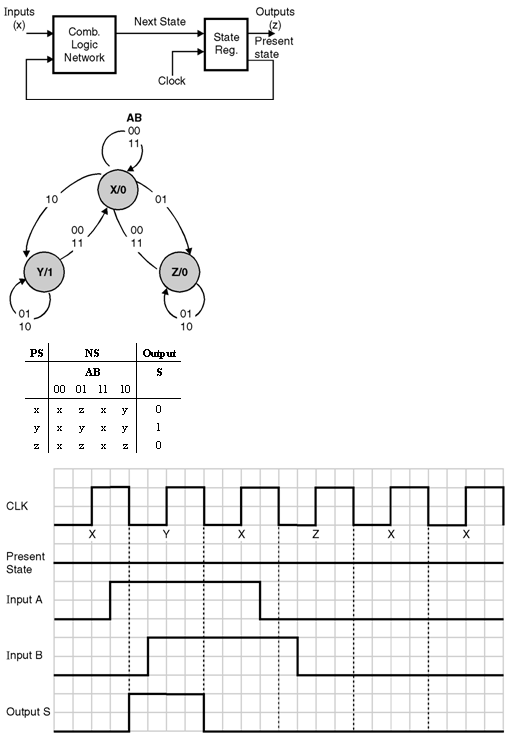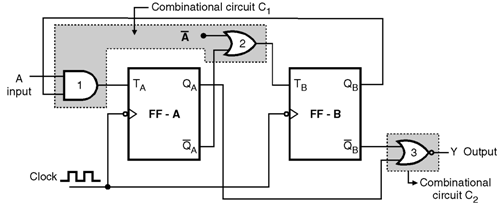Home > finite state machines > State Machine Fundamentals > Moore Finite State Machine

Moore Finite State Machine :

Moore Finite State Machine :

In Moore state machine sequential network the output is function of only present states. In Moore FSM the output is associated with the state, as is shown in the of Figure. In Moore FSM output is shown inside the state, since the output is same as the state machine is in that state. In Moore machine, the combination is shown by a the internal state. The Moore FSM is faster. The output can be arbitrarily complex but must be the same every time the machine enters that state. Moore machine realization is more complex than Mealy due to additional state requirements to derive the required outputs. Outputs of a Moore machine are generally robust and independent of external (primary) inputs.Example of Moore Circuit :

The example of a Moore circuit is shown in Figure below. Flip flops A and B act as the memory elements for the circuit. Gates 1 and 2 form the input combinational circuit C1, because the external inputs A and â€“A are applied to one of their inputs, where as the other inputs are coming from the QB and â€“QA outputs of the memory elements.Gate-3 acts as the output decoder or combinational circuit C2. The inputs to this gate are from the outputs of the two memory elements FF-A and FF-B.

ïœ Y = â€“â€“â€“â€“â€“â€“â€“ QA + â€“QB

Both the flip flops are negative edge triggered and the clock input is applied to both the flip flops simultaneously. Therefore the output Y will change only after the application of clock pulse.